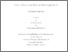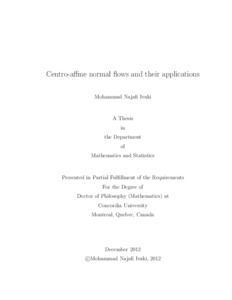Title:

# Centro-affine normal flows and their applications

Najafi Ivaki, Mohammad (2012) Centro-affine normal flows and their applications. PhD thesis, Concordia University.Preview Text (application/pdf) Ivaki_PhD_S2013.pdf - Accepted Version 604kB

## Abstract

A family of geometric flows, $p$ centro-affine normal flows, is studied and several applications of this family to convex geometry are presented. In Chapter 2, asymptotic behavior of the centro-affine normal flows is studied in the class of smooth, origin-symmetric convex bodies in $\mathbb{R}^2$. It is proved that the area preserving centro-affine normal flow evolve any smooth, origin-symmetric convex body to the unit disk in a finite time in the Hausdorff distance, module $SL(2).$ In Chapter 3, an application of centro-affine normal flow is given to the $L_{-2}$ Minkowski problem. It is proved there that every even, smooth, positive function can be approached by a sequence of functions for which the $L_{-2}$ Minkowski problem is solvable. In Chapter 4, another application of the centro-affine normal flows is given to the stability of the $p$-affine isoperimetric inequalities, $p\geq1.$ In Chapter 5, we end our study of the $p$ centro-affine normal flows in dimension two by classifying compact, origin-symmetric, ancient solutions to these flows for $1\leq p< 4$. In particular, we classify
origin-symmetric, compact ancient solutions of the planar affine normal flow. In the last chapter, we study the long time behavior of the $p$ centro-affine normal flows in $\mathbb{R}^n$ for $n>2$ and $1\leq p<\frac{n}{n-2}.$

Divisions: Concordia University > Faculty of Arts and Science > Mathematics and Statistics Thesis (PhD) Najafi Ivaki, Mohammad Concordia University Ph. D. Mathematics 3 December 2012 Stancu, Alina 977012 MOHAMMAD NAJAFI IVAKI 17 Apr 2013 13:57 18 Jan 2018 17:43
All items in Spectrum are protected by copyright, with all rights reserved. The use of items is governed by Spectrum's terms of access.

Repository Staff Only: item control pageResearch related to the current document (at the CORE website)
Back to top